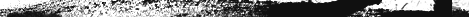G A T E F O R S T U D E N T S - C-aptitude (121-178)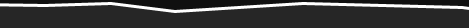121) void main()          { if(~0 == (unsigned int)-1) printf(“You can answer this if you know how values are represented in memory”);          }  Answer You can answer this if you know how values are represented in memory Explanation ~ (tilde operator or bit-wise negation operator) operates on 0 to produce all ones to fill the space for an integer. –1 is represented in unsigned value as all 1’s and so both are equal.   122) int swap(int *a,int *b) {  *a=*a+*b;*b=*a-*b;*a=*a-*b; } main() {                         int x=10,y=20;             swap(&x,&y);                         printf("x= %d y = %dn",x,y); } Answer             x = 20 y = 10 Explanation This is one way of swapping two values. Simple checking will help understand this.   123)     main() {          char *p = “ayqm”; printf(“%c”,++*(p++)); } Answer: b            124)     main()             {              int i=5;              printf("%d",++i++); } Answer:                         Compiler error: Lvalue required in function main Explanation:                         ++i yields an rvalue. For postfix ++ to operate an lvalue is required.   125)     main() { char *p = “ayqm”; char c; c = ++*p++; printf(“%c”,c); } Answer: b Explanation: There is no difference between the expression ++*(p++) and ++*p++. Parenthesis just works as a visual clue for the reader to see which expression is first evaluated.   126) int aaa() {printf(“Hi”);} int bbb(){printf(“hello”);} iny ccc(){printf(“bye”);}   main() { int ( * ptr) (); ptr = aaa; ptr = bbb; ptr =ccc; ptr(); } Answer:  bye Explanation: int (* ptr)() says that ptr is an array of pointers to functions that takes no arguments and returns the type int. By the assignment ptr = aaa; it means that the first function pointer in the array is initialized with the address of the function aaa. Similarly, the other two array elements also get initialized with the addresses of the functions bbb and ccc. Since ptr contains the address of the function ccc, the call to the function ptr() is same as calling ccc(). So it results in printing "bye".   127) main() { int i=5; printf(“%d”,i=++i ==6); }   Answer: 1 Explanation: The expression can be treated as i = (++i==6), because == is of higher precedence than = operator. In the inner expression, ++i is equal to 6 yielding true(1). Hence the result.   128)     main() {                         char p[ ]="%dn"; p = 'c'; printf(p,65); } Answer: A Explanation: Due to the assignment p = ‘c’ the string becomes, “%cn”. Since this string becomes the format string for printf and ASCII value of 65 is ‘A’, the same gets printed.   129)     void ( * abc( int, void ( *def) () ) ) ();   Answer::  abc is a ptr to a function which takes 2 parameters .(a). an integer variable.(b).        a ptrto a funtion which returns void. the return type of the function is void. Explanation:                         Apply the clock-wise rule to find the result.     130)     main() { while (strcmp(“some”,”some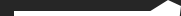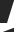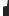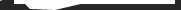Site Maintained by Saravanan.R.R. NithinRaj.PFor Job Searching www.naukri.com www.freshersworld.com www.jobsearch.monsterindia.com www.recruit.net www.timesjobs.com www.jobsearchworld.com www.placementindia.com www.bestjobsindia.in www.jobsahead.com www.jobsearch.co.in www.jobsearchinformation.com www.jobconsultancy.com www.marketingcrossing.com www.mobile.monsterindia.com www.hinduonnet.comTutorials Searching www.howstuffworks.com www.tutor.com www.en.wikipedia.org www.mathguru.com www.knowledgeonlineservices.com www.mygretutor.comEnglish Tutor Searching www.englishgrammartutor.com www.theenglishtutor.com www.learnenglish.de www.tolearnenglish.com www.englishclub.com www.english-at-home.com www.learn-english.co.il www.learnenglish.beFor Entrance Exam Details Searching www.winentrance.com www.entranceexamindia.com www.studyplaces.com www.entranceexam.info www.indiastudychannel.com www.edudiary.com www.successcds.net